# Texas Go Math Grade 3 Lesson 3.3 Answer Key Compare Fractions

Refer to our Texas Go Math Grade 3 Answer Key Pdf to score good marks in the exams. Test yourself by practicing the problems from Texas Go Math Grade 3 Lesson 3.3 Answer Key Compare Fractions.

## Texas Go Math Grade 3 Lesson 3.3 Answer Key Compare Fractions

Essential Question
What strategies can you use to compare fractions?
Equivalence can be used to make fractions easier to compare. For instance, to use the common denominator strategy to compare $$\frac{2}{5}$$ and $$\frac{3}{8}$$, each fraction can be rewritten as an equivalent fraction with a denominator of 40. Once the denominators are the same, one can simply compare the size of the resulting numerators

Unlock the Problem

Example 1
Molly and Omar are decorating the same-size bookmarks. Molly covers $$\frac{2}{3}$$ other bookmark with glitter. Omar covers $$\frac{1}{3}$$ of his bookmark with glitter. Whose bookmark is covered with more glitter?

• Do you need to look at the numerators or the denominators?
It is given that
Molly and Omar are decorating the same-size bookmarks. Molly covers $$\frac{2}{3}$$ other bookmark with glitter. Omar covers $$\frac{1}{3}$$ of his bookmark with glitter
Now,
The given fractions are:
$$\frac{2}{3}$$ and $$\frac{1}{3}$$
Hence, from the above,
We can conclude that
We need to look at the numerators since the denominators are the same

Use reasoning to compare $$\frac{2}{3}$$ and $$\frac{1}{3}$$.

• Both fractions involve third-size pieces. 2 pieces are more than 1 piece.
•So, Molly’s bookmark is covered with more glitter.

Same Denominator Strategy

• When the denominators are the same, you can compare only the number of pieces, or the numerators.

Example 2
Ms. Davis is making a fruit salad with $$\frac{3}{4}$$ pound of cherries and $$\frac{3}{8}$$ pound of strawberries. Which weighs less, the cherries or the strawberries?

Use reasoning to compare $$\frac{3}{4}$$ and $$\frac{3}{8}$$.
• $$\frac{1}{8}$$ is less than $$\frac{1}{4}$$ because there are more pieces.Same Numerator Strategy
• When the numerators are the same, look at the denominators to compare the size of the pieces.
So,
The strawberries weigh less.

Share and Show

Question 1.
Compare $$\frac{5}{8}$$ and $$\frac{5}{6}$$.
Think The more pieces a whole is divided into, the smaller the pieces are.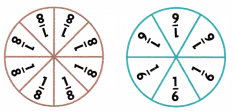Write <, >, or =. $$\frac{5}{8}$$$$\frac{5}{6}$$
The given fractions are:
$$\frac{5}{8}$$ and $$\frac{5}{6}$$
Now,
The representation of the given fractions in the models is:Now,
We know that,
The fraction with the biggest denominator is the smallest fraction when both the numerators are the same
So,
$$\frac{5}{8}$$ < $$\frac{5}{6}$$
Hence, from the above,
We can conclude thatMath Talk
Mathematical processes Explain how the denominators in Exercise 1 help you compare $$\frac{5}{8}$$ and $$\frac{5}{6}$$.
We know that,
The fraction with the biggest denominator is the smallest fraction when both the numerators are the same
Hence, from the above,
We can conclude that
$$\frac{5}{8}$$ < $$\frac{5}{6}$$

Question 2.
$$\frac{3}{8}$$$$\frac{3}{6}$$
The given fractions are:
$$\frac{3}{8}$$ and $$\frac{3}{6}$$
Now,
We know that,
The fraction with the biggest denominator is the smallest fraction when both the numerators are the same
Hence, from the above,
We can conclude that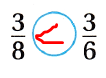$$\frac{3}{4}$$$$\frac{2}{4}$$
The given fractions are:
$$\frac{3}{4}$$ and $$\frac{2}{4}$$
Now,
We know that,
If the denominators are the same, then compare the numerators
Hence, from the above,
We can conclude that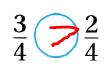Question 4.
$$\frac{5}{6}$$$$\frac{5}{6}$$
The given fractions are:
$$\frac{5}{6}$$ and $$\frac{5}{6}$$
Now,
We know that,
If the denominators are the same, then compare the numerators
Hence, from the above,
We can conclude that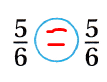Question 5.
$$\frac{4}{6}$$$$\frac{4}{2}$$
The given fractions are:
$$\frac{4}{6}$$ and $$\frac{4}{2}$$
Now,
We know that,
The fraction with the biggest denominator is the smallest fraction when both the numerators are the same
Hence, from the above,
We can conclude that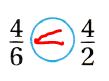Name a fraction that is less than or greater than the given fraction. Draw to justify your answer.

Question 6.
greater than $$\frac{2}{3}$$ ___
The given fraction is: $$\frac{2}{3}$$
Now,
We know that,
If the denominators are the same, then compare the numerators
The fraction with the biggest denominator is the smallest fraction when both the numerators are the same
Hence, from the above,
We can conclude that
The fraction that is greater than $$\frac{2}{3}$$ is: $$\frac{3}{3}$$
The fraction that is greater than $$\frac{2}{3}$$ is: $$\frac{2}{2}$$

Question 7.
less than $$\frac{6}{8}$$ ____
The given fraction is: $$\frac{6}{8}$$
Now,
We know that,
If the denominators are the same, then compare the numerators
The fraction with the biggest denominator is the smallest fraction when both the numerators are the same
Hence, from the above,
We can conclude that
The fraction that is less than $$\frac{6}{8}$$ is: $$\frac{5}{8}$$
The fraction that is less than $$\frac{6}{8}$$ is: $$\frac{6}{9}$$

Problem Solving

Question 8.
Apply In fifteen minutes, Serena’s sailboat went $$\frac{3}{6}$$ of a mile. Jeremy’s sailboat went $$\frac{3}{4}$$ of a mile. Whose sailboat went farther? Draw a picture to explain your answer.
It is given that
In fifteen minutes, Serena’s sailboat went $$\frac{3}{6}$$ of a mile. Jeremy’s sailboat went $$\frac{3}{4}$$ of a mile
Now,
The given fractions are:
$$\frac{3}{6}$$ and $$\frac{3}{4}$$
Now,
We know that,
The fraction with the biggest denominator is the smallest fraction when both the numerators are the same
So,
$$\frac{3}{6}$$ < $$\frac{3}{4}$$
Hence, from the above,
We can conclude that
Jeremy’s sailboat went farther

Unlock the Problem

Question 9.
H.O.T. Tracy is making a blueberry cake. She is using $$\frac{4}{4}$$ cup of sugar and $$\frac{4}{2}$$ cups of flour. Which of the following correctly compares the fractions?
(A) $$\frac{4}{4}$$ = $$\frac{4}{2}$$
(B) $$\frac{4}{4}$$ > $$\frac{4}{2}$$
(C) $$\frac{4}{2}$$ > $$\frac{4}{4}$$
(D) $$\frac{4}{2}$$ = $$\frac{4}{4}$$

a. What do you need to know? _________
It is given that
Tracy is making a blueberry cake. She is using $$\frac{4}{4}$$ cup of sugar and $$\frac{4}{2}$$ cups of flour
Hence, from the above,
We can conclude that
You need to know the greater number of cups of flour from the given cups of flour

b. What plan or strategy will you use to compare the fractions?
It is given that
Tracy is making a blueberry cake. She is using $$\frac{4}{4}$$ cup of sugar and $$\frac{4}{2}$$ cups of flour
Hence, from the above,
We can conclude that
The strategy we will use to compare the given fractions are:
The fraction with the biggest denominator is the smallest fraction when both the numerators are the same

c. Show the steps you used to solve the problem.
It is given that
Tracy is making a blueberry cake. She is using $$\frac{4}{4}$$ cup of sugar and $$\frac{4}{2}$$ cups of flour
Now,
The given fractions are:
$$\frac{4}{4}$$ and $$\frac{4}{2}$$
Now,
We know that,
The fraction with the biggest denominator is the smallest fraction when both the numerators are the same
Hence, from the above,
We can conclude that
$$\frac{4}{4}$$ < $$\frac{4}{2}$$

d. Complete the comparison.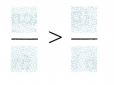The given fractions are:
$$\frac{4}{4}$$ and $$\frac{4}{2}$$
Hence, from the above,
We can conclude thate. Fill in the bubble for the correct answer choice above.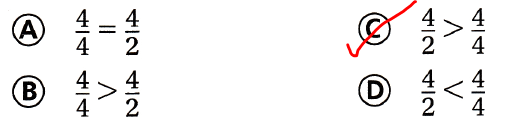Question 10.
Chloe is frosting 8 cupcakes for a party. Three-eighths of the cupcakes have vanilla frosting, $$\frac{4}{8}$$ have chocolate frosting, and $$\frac{1}{8}$$ have strawberry frosting. Which flavor frosting did Chloe use on the most cupcakes? Tell me which strategy you used.
It is given that
Chloe is frosting 8 cupcakes for a party. Three-eighths of the cupcakes have vanilla frosting, $$\frac{4}{8}$$ have chocolate frosting, and $$\frac{1}{8}$$ have strawberry frosting.
Now,
The given fractions are:
$$\frac{3}{8}$$, $$\frac{4}{8}$$, and $$\frac{1}{8}$$
Now,
We know that,
If the denominators are the same, then compare the numerators
So,
The order of the fractions is:
$$\frac{4}{8}$$ > $$\frac{3}{8}$$ > $$\frac{1}{8}$$
Hence, from the above,
We can conclude that
The flavor frosting that Chloe used on most cupcakes is: Chocolate frosting

Question 11.
H.O.T. Multi-Step Jack says that $$\frac{5}{8}$$ is greater than $$\frac{5}{6}$$ because the denominator 8 is greater than the denominator 6. Describe Jack’s error. Draw a picture to explain your answer.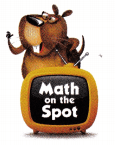It is given that
Jack says that $$\frac{5}{8}$$ is greater than $$\frac{5}{6}$$ because the denominator 8 is greater than the denominator 6.
Now,
The given fractions are:
$$\frac{5}{8}$$ and $$\frac{5}{6}$$
Now,
We know that,
The fraction with the biggest denominator will be the smallest fraction when the numerators of the given fractions are the same
So,
$$\frac{1}{8}$$ < $$\frac{1}{6}$$
Hence, from the above,
We can conclude that
Jack’s error is:
jack applied the strategy when the fractions are compared for the same denominator
$$\frac{5}{8}$$ < $$\frac{5}{6}$$

Use models to compare. Fifi In the bubble for the answer choice.

Question 12.
A banana is about $$\frac{6}{8}$$ water and an orange is about $$\frac{7}{8}$$ water. Which statement is correct?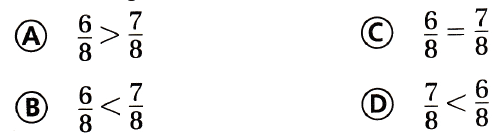It is given that
A banana is about $$\frac{6}{8}$$ water and an orange is about $$\frac{7}{8}$$ water.
Now,
The given fractions are:
$$\frac{6}{8}$$ and $$\frac{7}{8}$$
Now,
We know that,
If the denominators are the same, then compare the numerators
Hence, from the above,
We can conclude that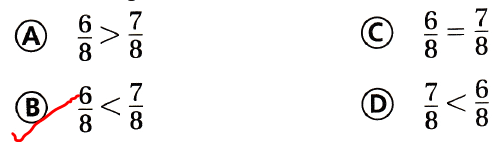Question 13.
Which symbol makes the statement true?(A) >
(B) <
(C) =
(D) none
The given fractions are:
$$\frac{4}{6}$$ and $$\frac{4}{8}$$
Now,
We know that,
The fraction with the biggest denominator will be the smallest fraction when the numerators are the same
Hence, from the above,
We can conclude that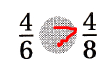Question 14.
Evaluate Multi-Step Jim loves fruit. He made a fruit salad using $$\frac{2}{4}$$ cup of grapes, $$\frac{3}{4}$$ cup of sliced strawberries, $$\frac{3}{4}$$ cup of chopped banana, and $$\frac{1}{4}$$ cup of chopped apple. Which fruit in Jim’s recipe is the least amount?
(A) grapes
(B) strawberries
(C) banana
(D) apple
It is given that
Jim loves fruit. He made a fruit salad using $$\frac{2}{4}$$ cup of grapes, $$\frac{3}{4}$$ cup of sliced strawberries, $$\frac{3}{4}$$ cup of chopped banana, and $$\frac{1}{4}$$ cup of chopped apple
Now,
The given fractions are:
$$\frac{2}{4}$$, $$\frac{3}{4}$$, $$\frac{3}{4}$$, and $$\frac{1}{4}$$
Now,
We know that,
If the denominators of the fractions are the same, then compare the denominators
So,
The order of the fractions is:
$$\frac{3}{4}$$ = $$\frac{3}{4}$$ > $$\frac{2}{4}$$ > $$\frac{1}{4}$$
Hence, from the above,
We can conclude that
The fruit in Jim’s recipe that is the least amount is: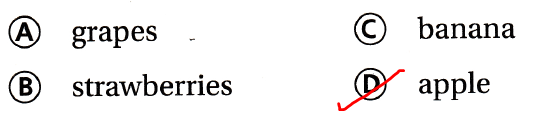Texas Test Prep

Question 15.
Luke and Seth have empty glasses. Mr. Gabel pours $$\frac{3}{4}$$ cup of orange juice in Seth’s glass. Then he pours $$\frac{1}{4}$$ cup of orange juice in Luke’s glass. Which statement is correct?
(A) Luke has less orange juice than Seth.
(B) Luke has more orange juice than Seth.
(C) Seth has less orange juice than Luke.
(D) Luke and Seth have the same amount of orange juice.
It is given that
Luke and Seth have empty glasses. Mr. Gabel pours $$\frac{3}{4}$$ cup of orange juice in Seth’s glass. Then he pours $$\frac{1}{4}$$ cup of orange juice in Luke’s glass
Now,
The given fractions are:
$$\frac{3}{4}$$ and $$\frac{1}{4}$$
Now,
We know that,
If the denominators are the same, then compare the numerators
So,
$$\frac{3}{4}$$ > $$\frac{1}{4}$$
Hence, from the above,
We can conclude that
The statement that is correct is: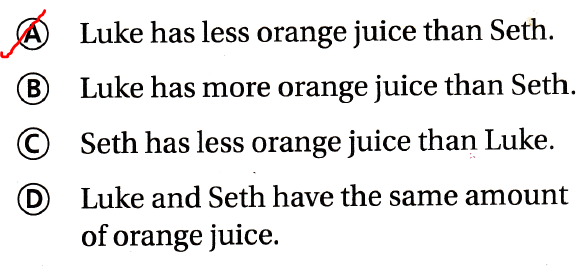### Texas Go Math Grade 3 Lesson 3.3 Homework and Practice Answer Key

Compare. Write <, >, or =. Write the strategy you used.

Question 1.
$$\frac{1}{3}$$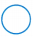$$\frac{1}{4}$$
The given fractions are:
$$\frac{1}{3}$$ and $$\frac{1}{4}$$
Now,
We know that,
The fraction with the biggest denominator will be the smallest fraction when the numerators are the same
Hence, from the above,
We can conclude that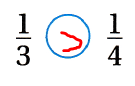Question 2.
$$\frac{2}{8}$$$$\frac{0}{8}$$
The given fractions are:
$$\frac{2}{8}$$ and $$\frac{0}{8}$$
Now,
We know that,
If the denominators of the fractions are the same, then compare the numerators
Hence, from the above,
We can conclude thatQuestion 3.
$$\frac{4}{6}$$$$\frac{4}{2}$$
The given fractions are:
$$\frac{4}{6}$$ and $$\frac{4}{2}$$
Now,
We know that,
The fraction with the biggest denominator will be the smallest fraction when the numerators are the same
Hence, from the above,
We can conclude that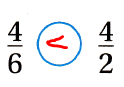Question 4.
$$\frac{1}{2}$$$$\frac{2}{2}$$
The given fractions are:
$$\frac{1}{2}$$ and $$\frac{2}{2}$$
Now,
We know that,
If the denominators of the fractions are the same, then compare the numerators
Hence, from the above,
We can conclude that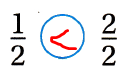Question 5.
$$\frac{5}{6}$$$$\frac{5}{6}$$
The given fractions are:
$$\frac{5}{6}$$ and $$\frac{5}{6}$$
Now,
We know that,
If the denominators of the fractions are the same, then compare the numerators
Hence, from the above,
We can conclude that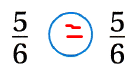Go Math Lesson 3.3 Homework Answer Key Question 6.
$$\frac{2}{3}$$$$\frac{1}{3}$$
The given fractions are:
$$\frac{2}{3}$$ and $$\frac{1}{3}$$
Now,
We know that,
If the denominators of the fractions are the same, then compare the numerators
Hence, from the above,
We can conclude that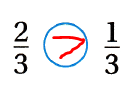Problem Solving

Question 7.
A player ran more than $$\frac{1}{3}$$ of the way down the field. Name a fraction that is more than $$\frac{1}{3}$$. Draw to justify your answer.
It is given that
A player ran more than $$\frac{1}{3}$$ of the way down the field
Now,
We know that,
If the denominators of the fractions are the same, then compare the numerators
The denominator that has the biggest number is the smallest fraction when the numerators are the same
Hence, from the above,
We can conclude that
The denominator that is greater than $$\frac{1}{3}$$ when the denominators are the same is: $$\frac{2}{3}$$
The denominator that is greater than $$\frac{1}{3}$$ when the numerators are the same is: $$\frac{1}{2}$$

Texas Test Prep

Lesson Check

Question 8.
Which symbol makes this statement true?(A) < (B) >
(C) =
(D) none
The given fractions are:
$$\frac{5}{8}$$ and $$\frac{6}{8}$$
Now,
We know that,
If the denominators of the fractions are the same, then compare the numerators
Hence, from the above,
We can conclude that
The symbol that makes the given statement true is:Question 9.
Which symbol makes this statement true?(A) < (B) >
(C) =
(D) none
The given fractions are:
$$\frac{2}{4}$$ and $$\frac{2}{4}$$
Now,
We know that,
If the denominators of the fractions are the same, then compare the numerators
Hence, from the above,
We can conclude that
The symbol that makes the given statement true is: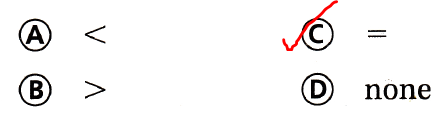Question 10.
A recipe for cake uses $$\frac{3}{3}$$ pound of blueberries and $$\frac{2}{3}$$ pound of apples. Which of the following correctly compares the fractions?
(A) $$\frac{2}{3}$$ > $$\frac{3}{3}$$
(B) $$\frac{3}{3}$$ < $$\frac{2}{3}$$ (C) $$\frac{3}{3}$$ > $$\frac{2}{3}$$
(D) $$\frac{2}{3}$$ = $$\frac{3}{3}$$
It is given that
A recipe for cake uses $$\frac{3}{3}$$ pound of blueberries and $$\frac{2}{3}$$ pound of apples.
Now,
The given fractions are:
$$\frac{3}{3}$$ and $$\frac{2}{3}$$
Now,
We know that,
If the denominators of the fractions are the same, then compare the numerators
So,
$$\frac{3}{3}$$ > $$\frac{2}{3}$$
Hence, from the above,
We can conclude that
The statement that correctly compares the fractions is:Use the recipe for 11-12.

Question 11.
Multi-Step Which food in the recipe is the least amount?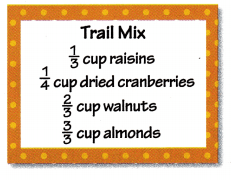(A) raisins
(B) cranberries
(C) walnuts
(D) almonds
The given table of cups of recipe is:Now,
From the above table,
The given fractions are:
$$\frac{1}{3}$$, $$\frac{1}{4}$$, $$\frac{2}{3}$$, and $$\frac{3}{3}$$
So,
The order of the given fractions are:
$$\frac{3}{3}$$ > $$\frac{2}{3}$$ > $$\frac{1}{3}$$ > $$\frac{1}{4}$$
Hence, from the above,
We can conclude that
The food in the recipe that is the least amount is: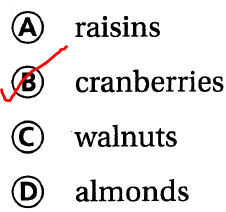Question 12.
Multi-Step Which food in the recipe is the greatest amount?
(A) raisins
(B) cranberries
(C) walnuts
(D) almonds
The given table of cups of recipe is:Now,
From the above table,
The given fractions are:
$$\frac{1}{3}$$, $$\frac{1}{4}$$, $$\frac{2}{3}$$, and $$\frac{3}{3}$$
So,
The order of the given fractions are:
$$\frac{3}{3}$$ > $$\frac{2}{3}$$ > $$\frac{1}{3}$$ > $$\frac{1}{4}$$
Hence, from the above,
We can conclude that
The food in the recipe that is the greatest amount is:Scroll to Top